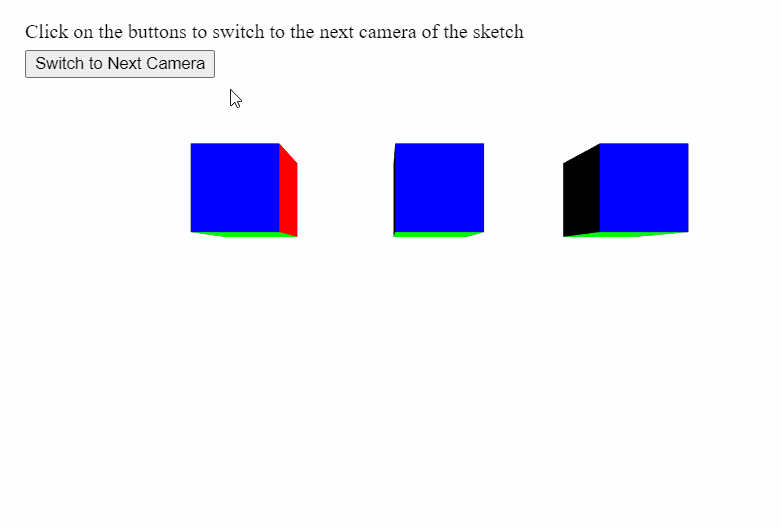Open in App
Not now

# p5.js | setCamera() Function

• Last Updated : 31 May, 2020

The setCamera() function in p5.js is used to set the renderer’s current camera to the given p5.Camera object. This can be used to switch to multiple cameras.

Syntax:

```setCamera( cam )
```

Parameters: This function accept a single parameter as mentioned above and described below:

• cam: It is a p5.Camera object that the function will change the camera to.

The example below illustrates the setCamera() function in p5.js:
Example:

## javascript

 `let cameras = []; ` `let currCameraIndex = 0; ` ` `  `function` `setup() { ` `  ``createCanvas(600, 400, WEBGL); ` `  ``helpText = createP( ` `    ``"Click on the buttons to switch to the"``+ ` `    ``" next camera of the sketch"` `  ``); ` `  ``helpText.position(20, 0); ` ` `  `  ``// Button to switch to the next camera ` `  ``// in the scene ` `  ``newCameraBtn = createButton(``"Switch to Next Camera"``); ` `  ``newCameraBtn.position(20, 40); ` `  ``newCameraBtn.mouseClicked(switchActiveCamera); ` ` `  `  ``// Create 5 cameras and store into array ` `  ``for` `(let i = 0; i < 5; i++) { ` `    ``cameras[i] = createCamera(); ` ` `  `    ``// Randomly set the position the camera ` `    ``// is looking at using setPosition() ` `    ``randomX = floor(random(-100, 100)); ` `    ``randomY = floor(random(-100, 100)); ` ` `  `    ``cameras[i].setPosition(randomX, randomY, 350); ` `  ``} ` `} ` ` `  `function` `switchActiveCamera() { ` `  ``// Increment the camera index ` `  ``if` `(currCameraIndex < 4) currCameraIndex += 1; ` `  ``else` `currCameraIndex = 0; ` ` `  `  ``// Set the camera from the camera array ` `  ``// to that index ` `  ``setCamera(cameras[currCameraIndex]); ` `} ` ` `  `function` `draw() { ` `  ``clear(); ` `  ``orbitControl(); ` `  ``normalMaterial(); ` ` `  `  ``// Create three boxes at three positions ` `  ``translate(-150, 0); ` `  ``box(65); ` `  ``translate(150, 0); ` `  ``box(65); ` `  ``translate(150, 0); ` `  ``box(65); ` `} `

Output:Online editor: https://editor.p5js.org/
Environment Setup: https://www.geeksforgeeks.org/p5-js-soundfile-object-installation-and-methods/
Reference: https://p5js.org/reference/#/p5/setCamera

My Personal Notes arrow_drop_up
Related Articles